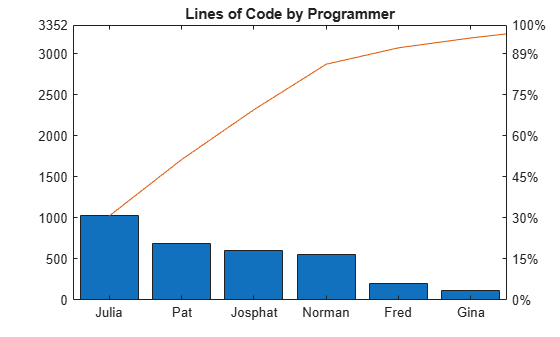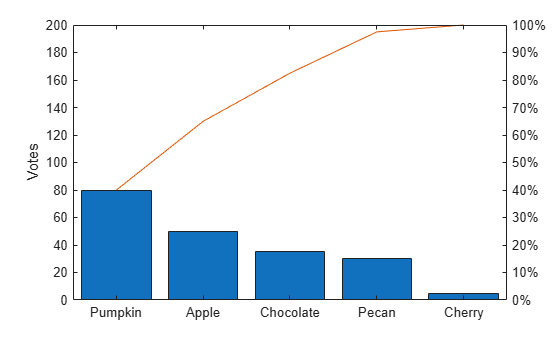Main Content

# pareto## Syntax

```pareto(Y) pareto(Y,names) pareto(Y,X) pareto(ax,..) H = pareto(...) [H,ax] = pareto(...) ```

## Description

Pareto charts display the values in the vector `Y` as bars drawn in descending order. Values in `Y` must be nonnegative and not include `NaN`s. By default, either the tallest 10 bars or first 95% of the cumulative distribution is displayed, whichever is smaller.

`pareto(Y)` labels each bar with its element index in `Y` and also plots a line displaying the cumulative sum of `Y`.

`pareto(Y,names)` labels each bar with the associated text in the matrix or cell array `names`.

`pareto(Y,X)` labels each bar with the associated value from `X`.

`pareto(ax,..)` plots into the axes `ax` rather than the current axes, `gca`.

`pareto(...,threshold)` specifies a threshold value between `0` and `1`. The threshold is the fraction of the cumulative histogram to include in the chart. The chart displays a maximum of ten bars, regardless of the threshold value.

`H = pareto(...)` returns the primitive `Line` and `Bar` objects created.

`[H,ax] = pareto(...)` additionally returns the two axes objects created.

## Examples

collapse all

Create a Pareto chart of vector `y`.

```y = [90,75,30,60,5,40,40,5]; figure pareto(y)````pareto` displays the elements in `y` as bars in descending order and labels each bar with its index in `y`. Since `pareto` displays only the first 95% of the cumulative distribution, some elements in `y` are not displayed.

Examine the cumulative productivity of a group of programmers to see how normal its distribution is. Label each bar with the name of the programmer.

```codelines = [200 120 555 608 1024 101 57 687]; coders = {'Fred','Ginger','Norman','Max','Julia','Wally','Heidi','Pat'}; figure pareto(codelines, coders) title('Lines of Code by Programmer')```Create a Pareto chart that examines the preferred types of pie in a survey of 200 participants. Include all the values in the cumulative distribution by setting the `threshold` argument to `1`.

```pies = {'Chocolate','Apple','Pecan','Cherry','Pumpkin'}; votes = [35 50 30 5 80]; pareto(votes,pies,1) ylabel('Votes')```## See Also

### Properties

Introduced before R2006a

Download ebook